# The Parabigyrate Rhombicosidodecahedron

The parabigyrate rhombicosidodecahedron is the 73rd Johnson solid, or J73 for short. It has 62 faces (12 pentagons, 30 squares, 20 triangles), 120 edges, and 60 vertices, just like the rhombicosidodecahedron, except that two opposite pentagonal cupola (J5) segments have been gyrated so that the polyhedron is no longer vertex-transitive.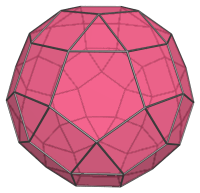J73 closely resembles the uniform rhombicosidodecahedron, but, just like J72, it can be recognized by noting the tell-tale pairs of adjacent squares and the triangles that share an edge with a pentagon: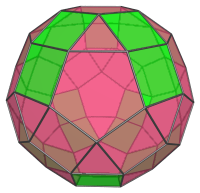The uniform rhombicosidodecahedron does not have square faces that share edges with each other, nor does it have triangles that share edges with pentagons. These features arise from the gyrated configuration of the pentagonal cupola segments highlighted below: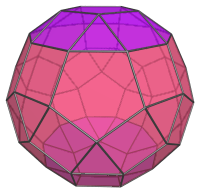J73 is somewhat more symmetrical than J72 because of the correspondence between the top and bottom gyrated cupola segments.

## Projections

Here are some views of the parabigyrate rhombicosidodecahedron from various angles:

Projection Description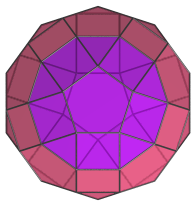Top view.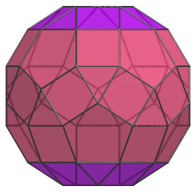Front view, parallel to pair of opposite triangles.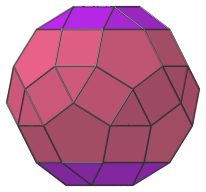Side view.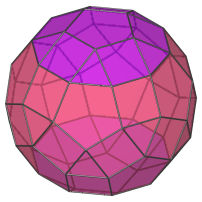Oblique view.

## Coordinates

The Cartesian coordinates of the parabigyrate rhombicosidodecahedron with edge length 2 are:

• (0, ±(φ+2), ±φ2)
• (±(φ+2), ±φ2, 0)
• 2, 0, −(φ+2))
• (−φ2, 0, (φ+2))
• (±1, ±1, ±φ3)
• (±1, ±φ3, ±1)
• 3, ±1, −1)
• (−φ3, ±1, 1)
• (±φ, ±φ2, ±2φ)
• (±φ2, ±2φ, ±φ)
• (2φ, ±φ, −φ2)
• (−2φ, ±φ, φ2)
• ±((8φ+1)/5, ±1, (6φ+7)/5)
• ±((8φ+6)/5, ±φ, (7+φ)/5)
• ±((13φ+1)/5, 0, (φ+2)/5)

where φ=(1+√5)/2 is the Golden Ratio.

Last updated 05 Apr 2019.